This is code that accompanies a book chapter on customer churn that I have written for the German dpunkt Verlag. The book is in German and will probably appear in February: https://www.dpunkt.de/buecher/13208/9783864906107-data-science.html.

The code you find below can be used to recreate all figures and analyses from this book chapter. Because the content is exclusively for the book, my descriptions around the code had to be minimal. But I’m sure, you can get the gist, even without the book. ;-)

# Inspiration & Sources

Thank you to the following people for providing excellent code examples about customer churn:

# Setup

All analyses are done in R using RStudio. For detailed session information including R version, operating system and package versions, see the `sessionInfo()` output at the end of this document.

All figures are produced with ggplot2.

• Libraries
``````# Load libraries
library(tidyverse) # for tidy data analysis
library(caret)     # for convenient splitting
library(mice)      # mice package for Multivariate Imputation by Chained Equations (MICE)
library(keras)     # for neural nets
library(lime)      # for explaining neural nets
library(rsample)   # for splitting training and test data
library(recipes)   # for preprocessing
library(yardstick) # for evaluation
library(ggthemes)  # for additional plotting themes
library(corrplot)  # for correlation

theme_set(theme_minimal())``````
``````# Install Keras if you have not installed it before
# follow instructions if you haven't installed TensorFlow
install_keras()``````

# Data preparation

## The dataset

The Telco Customer Churn data set is the same one that Matt Dancho used in his post (see above). It was downloaded from IBM Watson

``churn_data_raw <- read_csv("WA_Fn-UseC_-Telco-Customer-Churn.csv")``
``glimpse(churn_data_raw)``
``````## Observations: 7,043
## Variables: 21
## \$ customerID       <chr> "7590-VHVEG", "5575-GNVDE", "3668-QPYBK", "77...
## \$ gender           <chr> "Female", "Male", "Male", "Male", "Female", "...
## \$ SeniorCitizen    <dbl> 0, 0, 0, 0, 0, 0, 0, 0, 0, 0, 0, 0, 0, 0, 0, ...
## \$ Partner          <chr> "Yes", "No", "No", "No", "No", "No", "No", "N...
## \$ Dependents       <chr> "No", "No", "No", "No", "No", "No", "Yes", "N...
## \$ tenure           <dbl> 1, 34, 2, 45, 2, 8, 22, 10, 28, 62, 13, 16, 5...
## \$ PhoneService     <chr> "No", "Yes", "Yes", "No", "Yes", "Yes", "Yes"...
## \$ MultipleLines    <chr> "No phone service", "No", "No", "No phone ser...
## \$ InternetService  <chr> "DSL", "DSL", "DSL", "DSL", "Fiber optic", "F...
## \$ OnlineSecurity   <chr> "No", "Yes", "Yes", "Yes", "No", "No", "No", ...
## \$ OnlineBackup     <chr> "Yes", "No", "Yes", "No", "No", "No", "Yes", ...
## \$ DeviceProtection <chr> "No", "Yes", "No", "Yes", "No", "Yes", "No", ...
## \$ TechSupport      <chr> "No", "No", "No", "Yes", "No", "No", "No", "N...
## \$ StreamingTV      <chr> "No", "No", "No", "No", "No", "Yes", "Yes", "...
## \$ StreamingMovies  <chr> "No", "No", "No", "No", "No", "Yes", "No", "N...
## \$ Contract         <chr> "Month-to-month", "One year", "Month-to-month...
## \$ PaperlessBilling <chr> "Yes", "No", "Yes", "No", "Yes", "Yes", "Yes"...
## \$ PaymentMethod    <chr> "Electronic check", "Mailed check", "Mailed c...
## \$ MonthlyCharges   <dbl> 29.85, 56.95, 53.85, 42.30, 70.70, 99.65, 89....
## \$ TotalCharges     <dbl> 29.85, 1889.50, 108.15, 1840.75, 151.65, 820....
## \$ Churn            <chr> "No", "No", "Yes", "No", "Yes", "Yes", "No", ...``````

### EDA

• Proportion of churn
``````churn_data_raw %>%
count(Churn)``````
``````## # A tibble: 2 x 2
##   Churn     n
##   <chr> <int>
## 1 No     5174
## 2 Yes    1869``````
• Plot categorical features
``````churn_data_raw %>%
mutate(SeniorCitizen = as.character(SeniorCitizen)) %>%
select(-customerID) %>%
select_if(is.character) %>%
select(Churn, everything()) %>%
gather(x, y, gender:PaymentMethod) %>%
count(Churn, x, y) %>%
ggplot(aes(x = y, y = n, fill = Churn, color = Churn)) +
facet_wrap(~ x, ncol = 4, scales = "free") +
geom_bar(stat = "identity", alpha = 0.5) +
theme(axis.text.x = element_text(angle = 90, hjust = 1),
legend.position = "top") +
scale_color_tableau() +
scale_fill_tableau()``````• Plot numerical features
``````churn_data_raw %>%
select(-customerID) %>%
#select_if(is.numeric) %>%
select(Churn, MonthlyCharges, tenure, TotalCharges) %>%
gather(x, y, MonthlyCharges:TotalCharges) %>%
ggplot(aes(x = y, fill = Churn, color = Churn)) +
facet_wrap(~ x, ncol = 3, scales = "free") +
geom_density(alpha = 0.5) +
theme(axis.text.x = element_text(angle = 90, hjust = 1),
legend.position = "top") +
scale_color_tableau() +
scale_fill_tableau()``````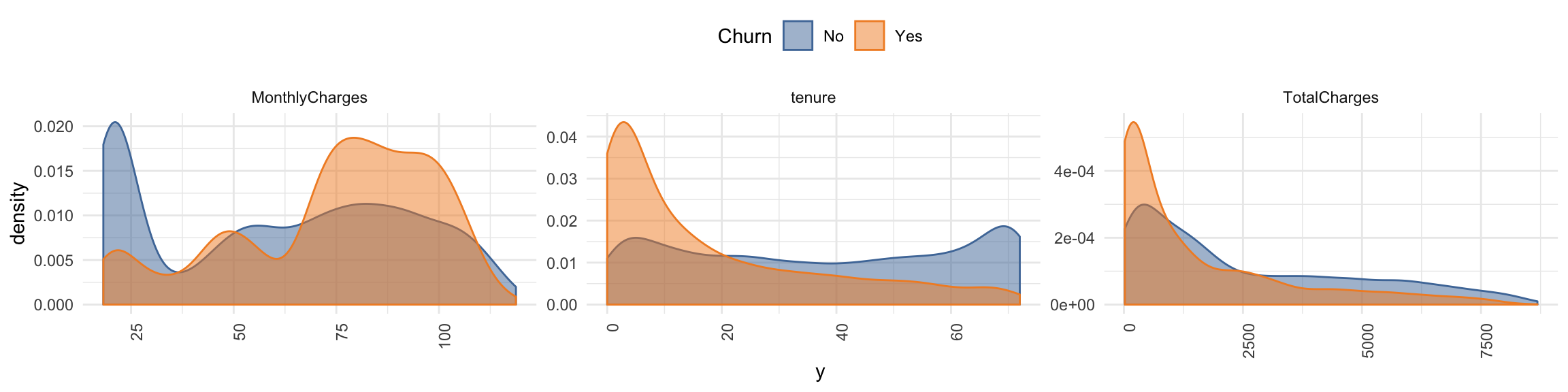• Remove customer ID as it doesn’t provide information
``````churn_data <- churn_data_raw %>%
select(-customerID)``````

### Dealing with missing values

• Pattern of missing data
``md.pattern(churn_data, plot = FALSE)``
``````##      gender SeniorCitizen Partner Dependents tenure PhoneService
## 7032      1             1       1          1      1            1
## 11        1             1       1          1      1            1
##           0             0       0          0      0            0
##      MultipleLines InternetService OnlineSecurity OnlineBackup
## 7032             1               1              1            1
## 11               1               1              1            1
##                  0               0              0            0
##      DeviceProtection TechSupport StreamingTV StreamingMovies Contract
## 7032                1           1           1               1        1
## 11                  1           1           1               1        1
##                     0           0           0               0        0
##      PaperlessBilling PaymentMethod MonthlyCharges Churn TotalCharges
## 7032                1             1              1     1            1  0
## 11                  1             1              1     1            0  1
##                     0             0              0     0           11 11``````
• Option 1: impute missing data => NOT done here!
``````imp <- mice(data = churn_data,  print = FALSE)
train_data_impute <- complete(imp, "long")``````
• Option 2: drop missing data => done here because not too much information is lost by removing it
``````churn_data <- churn_data %>%
drop_na()``````

## Training and test split

• Partition data into training and test set
``````set.seed(42)
index <- createDataPartition(churn_data\$Churn, p = 0.7, list = FALSE)``````
• Partition test set again into validation and test set
``````train_data <- churn_data[index, ]
test_data  <- churn_data[-index, ]

index2 <- createDataPartition(test_data\$Churn, p = 0.5, list = FALSE)

valid_data <- test_data[-index2, ]
test_data <- test_data[index2, ]``````
``nrow(train_data)``
``##  4924``
``nrow(valid_data)``
``##  1054``
``nrow(test_data)``
``##  1054``

## Pre-Processing

• Create recipe for preprocessing

A recipe is a description of what steps should be applied to a data set in order to get it ready for data analysis.

``````recipe_churn <- recipe(Churn ~ ., train_data) %>%
step_dummy(all_nominal(), -all_outcomes()) %>%
step_center(all_predictors(), -all_outcomes()) %>%
step_scale(all_predictors(), -all_outcomes()) %>%
prep(data = train_data)``````
• Apply recipe to three datasets
``````train_data <- bake(recipe_churn, new_data = train_data) %>%
select(Churn, everything())

valid_data <- bake(recipe_churn, new_data = valid_data) %>%
select(Churn, everything())

test_data <- bake(recipe_churn, new_data = test_data) %>%
select(Churn, everything())``````
• For Keras create response variable as one-hot encoded matrix
``````train_y_drop <- to_categorical(as.integer(as.factor(train_data\$Churn)) - 1, 2)
colnames(train_y_drop) <- c("No", "Yes")

valid_y_drop <- to_categorical(as.integer(as.factor(valid_data\$Churn)) - 1, 2)
colnames(valid_y_drop) <- c("No", "Yes")

test_y_drop <- to_categorical(as.integer(as.factor(test_data\$Churn)) - 1, 2)
colnames(test_y_drop) <- c("No", "Yes")``````
• Because we want to train on a binary outcome, we can delete the “No” column
``````# if training with binary crossentropy
train_y_drop <- train_y_drop[, 2, drop = FALSE]
``````##      Yes
## [1,]   0
## [2,]   1
## [3,]   1
## [4,]   0
## [5,]   1
## [6,]   0``````
``````valid_y_drop <- valid_y_drop[, 2, drop = FALSE]
test_y_drop <- test_y_drop[, 2, drop = FALSE]``````
• Remove response variable from preprocessed data (for Keras)
``````train_data_bk <- select(train_data, -Churn)
``````## # A tibble: 6 x 30
##   SeniorCitizen  tenure MonthlyCharges TotalCharges gender_Male Partner_Yes
##           <dbl>   <dbl>          <dbl>        <dbl>       <dbl>       <dbl>
## 1        -0.439  0.0765         -0.256       -0.163       0.979      -0.966
## 2        -0.439 -1.23           -0.359       -0.949       0.979      -0.966
## 3        -0.439 -0.983           1.17        -0.635      -1.02       -0.966
## 4        -0.439 -0.901          -1.16        -0.863      -1.02       -0.966
## 5        -0.439 -0.168           1.34         0.347      -1.02        1.03
## 6        -0.439  1.22           -0.282        0.542       0.979      -0.966
## # ... with 24 more variables: Dependents_Yes <dbl>,
## #   PhoneService_Yes <dbl>, MultipleLines_No.phone.service <dbl>,
## #   MultipleLines_Yes <dbl>, InternetService_Fiber.optic <dbl>,
## #   InternetService_No <dbl>, OnlineSecurity_No.internet.service <dbl>,
## #   OnlineSecurity_Yes <dbl>, OnlineBackup_No.internet.service <dbl>,
## #   OnlineBackup_Yes <dbl>, DeviceProtection_No.internet.service <dbl>,
## #   DeviceProtection_Yes <dbl>, TechSupport_No.internet.service <dbl>,
## #   TechSupport_Yes <dbl>, StreamingTV_No.internet.service <dbl>,
## #   StreamingTV_Yes <dbl>, StreamingMovies_No.internet.service <dbl>,
## #   StreamingMovies_Yes <dbl>, Contract_One.year <dbl>,
## #   Contract_Two.year <dbl>, PaperlessBilling_Yes <dbl>,
## #   PaymentMethod_Credit.card..automatic. <dbl>,
## #   PaymentMethod_Electronic.check <dbl>, PaymentMethod_Mailed.check <dbl>``````
``````valid_data_bk <- select(valid_data, -Churn)
test_data_bk <- select(test_data, -Churn)``````
• Alternative to above, to convert response variable into numeric format where 1 = Yes and 0 = No
``````train_data\$Churn <- ifelse(train_data\$Churn == "Yes", 1, 0)
valid_data\$Churn <- ifelse(valid_data\$Churn == "Yes", 1, 0)
test_data\$Churn <- ifelse(test_data\$Churn == "Yes", 1, 0)``````

## Modeling with Keras

• Define a simple MLP
``````model_keras <- keras_model_sequential()

model_keras %>%
layer_dense(units = 32, kernel_initializer = "uniform", activation = "relu",
input_shape = ncol(train_data_bk)) %>%
layer_dropout(rate = 0.2) %>%

layer_dense(units = 16, kernel_initializer = "uniform", activation = "relu") %>%
layer_dropout(rate = 0.2) %>%

layer_dense(units = 8, kernel_initializer = "uniform", activation = "relu") %>%
layer_dropout(rate = 0.2) %>%

layer_dense(units = 1,
kernel_initializer = "uniform", activation = "sigmoid") %>%

compile(
loss      = 'binary_crossentropy',
metrics   = c("binary_accuracy", "mse")
)

summary(model_keras)``````
``````## ___________________________________________________________________________
## Layer (type)                     Output Shape                  Param #
## ===========================================================================
## dense_1 (Dense)                  (None, 32)                    992
## ___________________________________________________________________________
## dropout_1 (Dropout)              (None, 32)                    0
## ___________________________________________________________________________
## dense_2 (Dense)                  (None, 16)                    528
## ___________________________________________________________________________
## dropout_2 (Dropout)              (None, 16)                    0
## ___________________________________________________________________________
## dense_3 (Dense)                  (None, 8)                     136
## ___________________________________________________________________________
## dropout_3 (Dropout)              (None, 8)                     0
## ___________________________________________________________________________
## dense_4 (Dense)                  (None, 1)                     9
## ===========================================================================
## Total params: 1,665
## Trainable params: 1,665
## Non-trainable params: 0
## ___________________________________________________________________________``````
• Fit model (we could have used validation split on the trainings data instead of creating a validation set => see #)
``````fit_keras <- fit(model_keras,
x = as.matrix(train_data_bk),
y = train_y_drop,
batch_size = 32,
epochs = 20,
#validation_split = 0.30,
validation_data = list(as.matrix(valid_data_bk), valid_y_drop),
verbose = 2
)``````
• Plot Keras training results
``````plot(fit_keras) +
scale_color_tableau() +
scale_fill_tableau()``````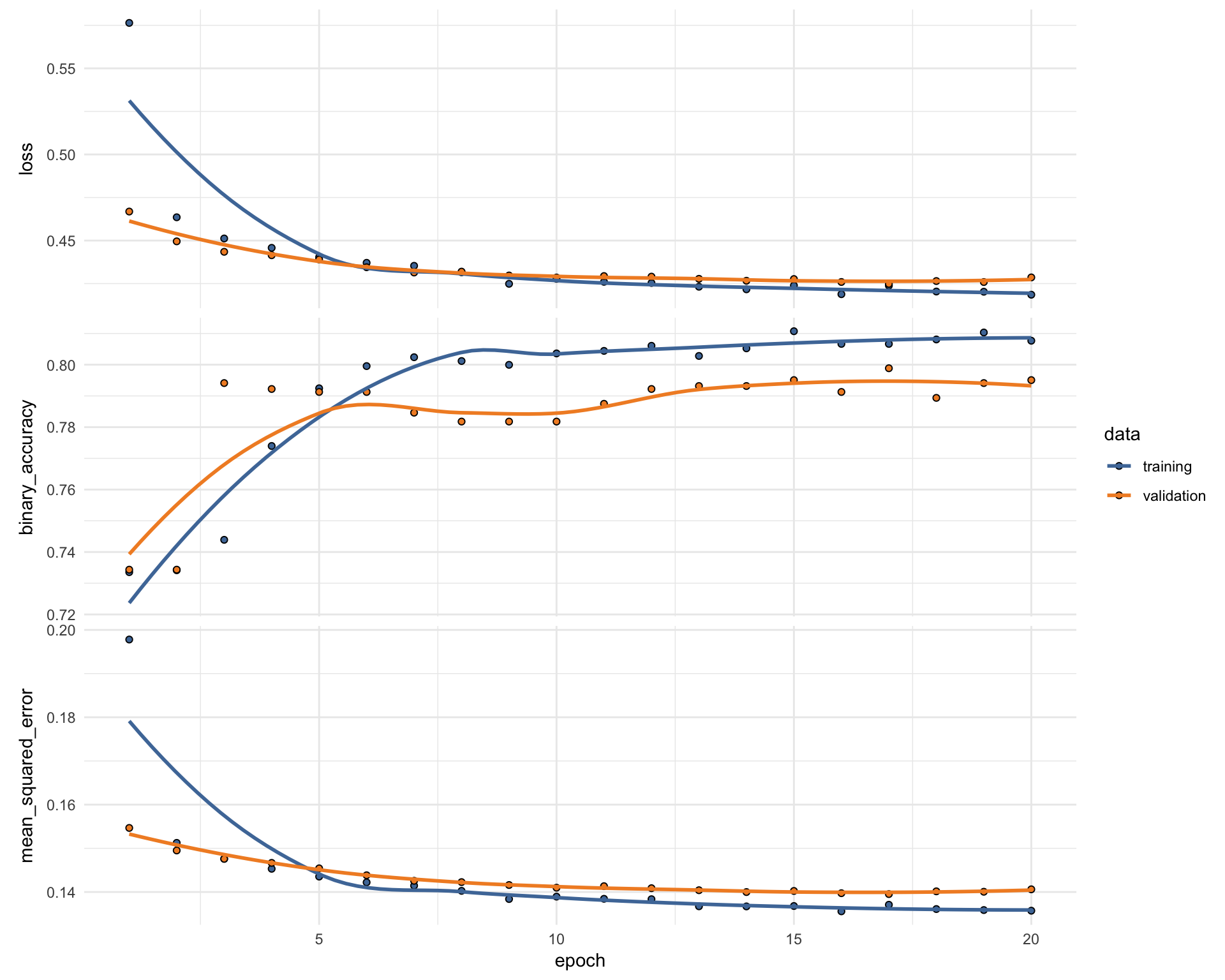## Evaluation

• Predict classes and probabilities
``````pred_classes_test <- predict_classes(object = model_keras, x = as.matrix(test_data_bk))
pred_proba_test  <- predict_proba(object = model_keras, x = as.matrix(test_data_bk))``````
• Create results table
``````test_results <- tibble(
actual_yes = as.factor(as.vector(test_y_drop)),
pred_classes_test = as.factor(as.vector(pred_classes_test)),
Yes = as.vector(pred_proba_test),
No = 1 - as.vector(pred_proba_test))
``````## # A tibble: 6 x 4
##   actual_yes pred_classes_test     Yes    No
##   <fct>      <fct>               <dbl> <dbl>
## 1 0          1                 0.516   0.484
## 2 0          0                 0.0145  0.985
## 3 1          1                 0.680   0.320
## 4 1          0                 0.390   0.610
## 5 0          0                 0.00610 0.994
## 6 0          0                 0.0371  0.963``````
• Calculate confusion matrix
``````test_results %>%
conf_mat(actual_yes, pred_classes_test)``````
``````##           Truth
## Prediction   0   1
##          0 697 128
##          1  77 152``````
• Calculate metrics
``````test_results %>%
metrics(actual_yes, pred_classes_test)``````
``````## # A tibble: 2 x 3
##   .metric  .estimator .estimate
##   <chr>    <chr>          <dbl>
## 1 accuracy binary         0.806
## 2 kap      binary         0.471``````
• Are under the ROC curve
``````test_results %>%
roc_auc(actual_yes, Yes)``````
``````## # A tibble: 1 x 3
##   .metric .estimator .estimate
##   <chr>   <chr>          <dbl>
## 1 roc_auc binary         0.840``````
• Precision and recall
``````tibble(
precision = test_results %>% yardstick::precision(actual_yes, pred_classes_test) %>% select(.estimate) %>% as.numeric(),
recall    = test_results %>% yardstick::recall(actual_yes, pred_classes_test) %>% select(.estimate) %>% as.numeric()
)``````
``````## # A tibble: 1 x 2
##   precision recall
##       <dbl>  <dbl>
## 1     0.845  0.901``````
• F1-Statistic
``test_results %>% yardstick::f_meas(actual_yes, pred_classes_test, beta = 1)``
``````## # A tibble: 1 x 3
##   .metric .estimator .estimate
##   <chr>   <chr>          <dbl>
## 1 f_meas  binary         0.872``````

## H2O

Shows an alternative to Keras!

• Initialise H2O instance and convert data to h2o frame
``````library(h2o)
``````##  Connection successful!
##
## R is connected to the H2O cluster:
##     H2O cluster uptime:         2 minutes 36 seconds
##     H2O cluster timezone:       Europe/Berlin
##     H2O data parsing timezone:  UTC
##     H2O cluster version:        3.20.0.8
##     H2O cluster version age:    3 months and 25 days !!!
##     H2O cluster name:           H2O_started_from_R_shiringlander_pir412
##     H2O cluster total nodes:    1
##     H2O cluster total memory:   3.47 GB
##     H2O cluster total cores:    8
##     H2O cluster allowed cores:  8
##     H2O cluster healthy:        TRUE
##     H2O Connection ip:          localhost
##     H2O Connection port:        54321
##     H2O Connection proxy:       NA
##     H2O Internal Security:      FALSE
##     H2O API Extensions:         XGBoost, Algos, AutoML, Core V3, Core V4
##     R Version:                  R version 3.5.1 (2018-07-02)``````
``````h2o.no_progress()

train_hf <- as.h2o(train_data)
valid_hf <- as.h2o(valid_data)
test_hf <- as.h2o(test_data)

response <- "Churn"
features <- setdiff(colnames(train_hf), response)``````
``````# For binary classification, response should be a factor
train_hf[, response] <- as.factor(train_hf[, response])
valid_hf[, response] <- as.factor(valid_hf[, response])
test_hf[, response] <- as.factor(test_hf[, response])``````
``summary(train_hf\$Churn, exact_quantiles = TRUE)``
``````##  Churn
##  0:3615
##  1:1309``````
``summary(valid_hf\$Churn, exact_quantiles = TRUE)``
``````##  Churn
##  0:774
##  1:280``````
``summary(test_hf\$Churn, exact_quantiles = TRUE)``
``````##  Churn
##  0:774
##  1:280``````

“During model training, you might find that the majority of your data belongs in a single class. For example, consider a binary classification model that has 100 rows, with 80 rows labeled as class 1 and the remaining 20 rows labeled as class 2. This is a common scenario, given that machine learning attempts to predict class 1 with the highest accuracy. It can also be an example of an imbalanced dataset, in this case, with a ratio of 4:1. The balance_classes option can be used to balance the class distribution. When enabled, H2O will either undersample the majority classes or oversample the minority classes. Note that the resulting model will also correct the final probabilities (“undo the sampling”) using a monotonic transform, so the predicted probabilities of the first model will differ from a second model. However, because AUC only cares about ordering, it won’t be affected. If this option is enabled, then you can also specify a value for the class_sampling_factors and max_after_balance_size options.” http://docs.h2o.ai/h2o/latest-stable/h2o-docs/data-science/algo-params/balance_classes.html

``````aml <- h2o.automl(x = features,
y = response,
training_frame = train_hf,
validation_frame = valid_hf,
balance_classes = TRUE,
max_runtime_secs = 3600)

h2o.saveModel(best_model, "/Users/shiringlander/Documents/Github/Data")``````
• Prediction
``pred <- h2o.predict(best_model, test_hf[, -1])``
• Mean per class error
``h2o.mean_per_class_error(best_model, train = TRUE, valid = TRUE, xval = TRUE)``
``````##     train     valid      xval
## 0.1717911 0.2172683 0.2350682``````
• Confusion matrix on validation data
``h2o.confusionMatrix(best_model, valid = TRUE)``
``````## Confusion Matrix (vertical: actual; across: predicted)  for max f1 @ threshold = 0.258586059604449:
##          0   1    Error      Rate
## 0      478 130 0.213816  =130/608
## 1       49 173 0.220721   =49/222
## Totals 527 303 0.215663  =179/830``````
``h2o.auc(best_model, train = TRUE)``
``##  0.9039696``
``h2o.auc(best_model, valid = TRUE)``
``##  0.8509068``
``h2o.auc(best_model, xval = TRUE)``
``##  0.8397085``
• Performance and confusion matrix on test data
``````perf <- h2o.performance(best_model, test_hf)
h2o.confusionMatrix(perf)``````
``````## Confusion Matrix (vertical: actual; across: predicted)  for max f1 @ threshold = 0.40790847476229:
##          0   1    Error       Rate
## 0      667 107 0.138243   =107/774
## 1       86 194 0.307143    =86/280
## Totals 753 301 0.183112  =193/1054``````
• Plot performance
``plot(perf)``
• More performance metrics extracted
``h2o.logloss(perf)``
``##  0.4008041``
``h2o.mse(perf)``
``##  0.1278505``
``h2o.auc(perf)``
``##  0.8622301``
``````metrics <- as.data.frame(h2o.metric(perf))
``````##   threshold         f1          f2   f0point5  accuracy precision
## 1 0.8278177 0.01418440 0.008912656 0.03472222 0.7362429         1
## 2 0.8203439 0.02816901 0.017793594 0.06756757 0.7381404         1
## 3 0.8173635 0.04195804 0.026642984 0.09868421 0.7400380         1
## 4 0.8160146 0.04878049 0.031055901 0.11363636 0.7409867         1
## 5 0.8139018 0.05555556 0.035460993 0.12820513 0.7419355         1
## 6 0.8112067 0.07560137 0.048629531 0.16975309 0.7447818         1
##        recall specificity absolute_mcc min_per_class_accuracy
## 1 0.007142857           1   0.07249342            0.007142857
## 2 0.014285714           1   0.10261877            0.014285714
## 3 0.021428571           1   0.12580168            0.021428571
## 4 0.025000000           1   0.13594622            0.025000000
## 5 0.028571429           1   0.14540208            0.028571429
## 6 0.039285714           1   0.17074408            0.039285714
##   mean_per_class_accuracy tns fns fps tps tnr       fnr fpr         tpr
## 1               0.5035714 774 278   0   2   1 0.9928571   0 0.007142857
## 2               0.5071429 774 276   0   4   1 0.9857143   0 0.014285714
## 3               0.5107143 774 274   0   6   1 0.9785714   0 0.021428571
## 4               0.5125000 774 273   0   7   1 0.9750000   0 0.025000000
## 5               0.5142857 774 272   0   8   1 0.9714286   0 0.028571429
## 6               0.5196429 774 269   0  11   1 0.9607143   0 0.039285714
##   idx
## 1   0
## 2   1
## 3   2
## 4   3
## 5   4
## 6   5``````
• Plot performance metrics
``````metrics %>%
gather(x, y, f1:tpr) %>%
ggplot(aes(x = threshold, y = y, group = x)) +
facet_wrap(~ x, ncol = 2, scales = "free") +
geom_line()``````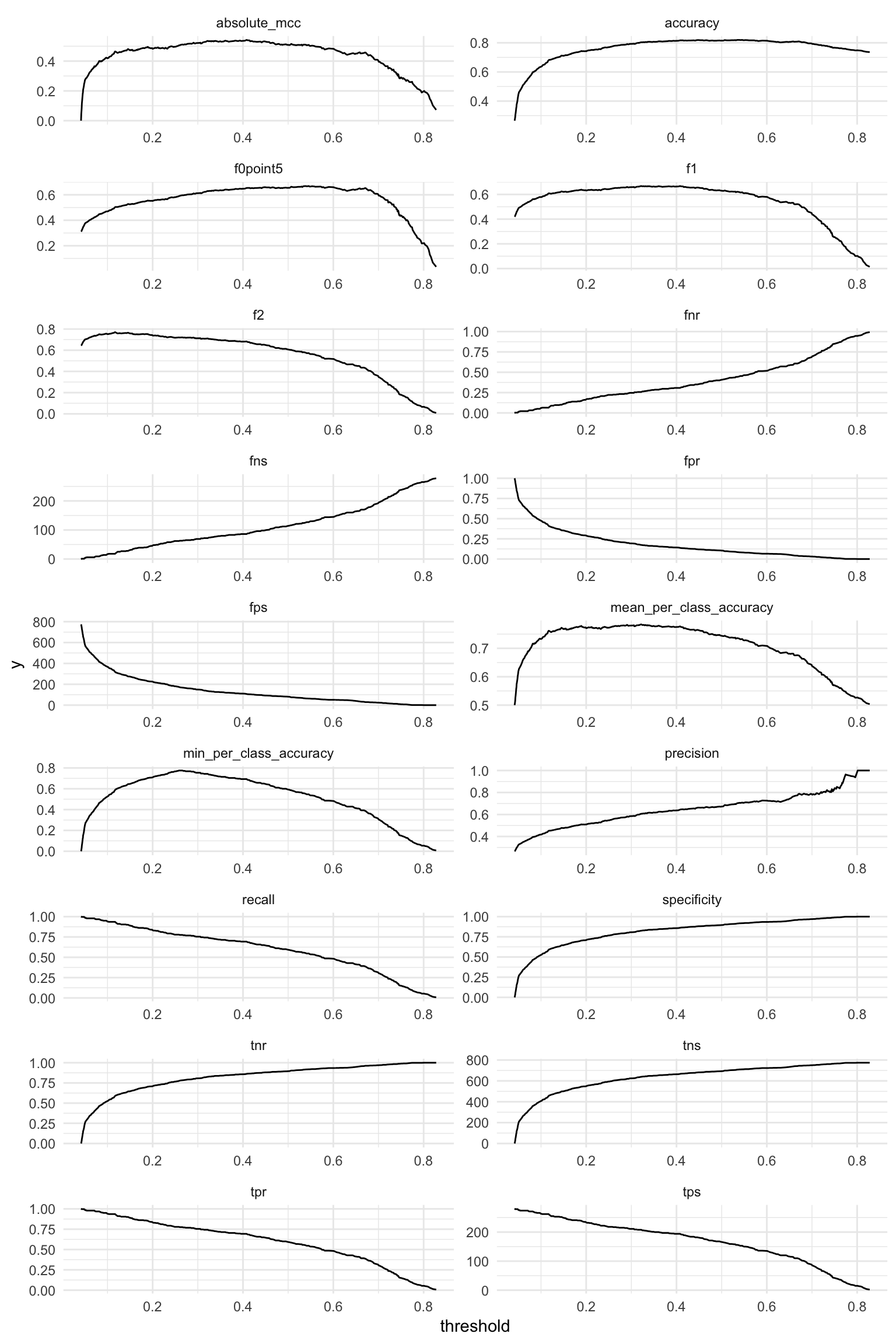• Examine prediction thresholds
``````threshold <- metrics[order(-metrics\$accuracy), "threshold"]

finalRf_predictions <- data.frame(actual = as.vector(test_hf\$Churn),
as.data.frame(h2o.predict(object = best_model,
newdata = test_hf)))
``````##   actual predict        p0         p1
## 1      0       1 0.3870607 0.61293929
## 2      0       0 0.9341973 0.06580274
## 3      1       1 0.2868668 0.71313316
## 4      1       1 0.3498788 0.65012119
## 5      0       0 0.9491335 0.05086646
## 6      0       0 0.9364659 0.06353410``````
``````finalRf_predictions\$accurate <- ifelse(finalRf_predictions\$actual ==
finalRf_predictions\$predict, "ja", "nein")

finalRf_predictions\$predict_stringent <- ifelse(finalRf_predictions\$p1 > threshold, 1,
ifelse(finalRf_predictions\$p0 > threshold, 0, "unsicher"))
finalRf_predictions\$accurate_stringent <- ifelse(finalRf_predictions\$actual ==
finalRf_predictions\$predict_stringent, "ja",
ifelse(finalRf_predictions\$predict_stringent ==
"unsicher", "unsicher", "nein"))

finalRf_predictions %>%
group_by(actual, predict) %>%
dplyr::summarise(n = n())``````
``````## # A tibble: 4 x 3
## # Groups:   actual [?]
##   actual predict     n
##   <fct>  <fct>   <int>
## 1 0      0         602
## 2 0      1         172
## 3 1      0          63
## 4 1      1         217``````
``````finalRf_predictions %>%
group_by(actual, predict_stringent) %>%
dplyr::summarise(n = n())``````
``````## # A tibble: 6 x 3
## # Groups:   actual [?]
##   actual predict_stringent     n
##   <fct>  <chr>             <int>
## 1 0      0                   683
## 2 0      1                    63
## 3 0      unsicher             28
## 4 1      0                   101
## 5 1      1                   152
## 6 1      unsicher             27``````
``````finalRf_predictions %>%
gather(x, y, accurate, accurate_stringent) %>%
mutate(x = ifelse(x == "accurate", "Default Schwelle: 0.5",
paste("Angepasste Schwelle:", round(threshold, digits = 2)))) %>%
ggplot(aes(x = actual, fill = y)) +
facet_grid(~ x) +
geom_bar(position = "dodge") +
scale_fill_tableau()``````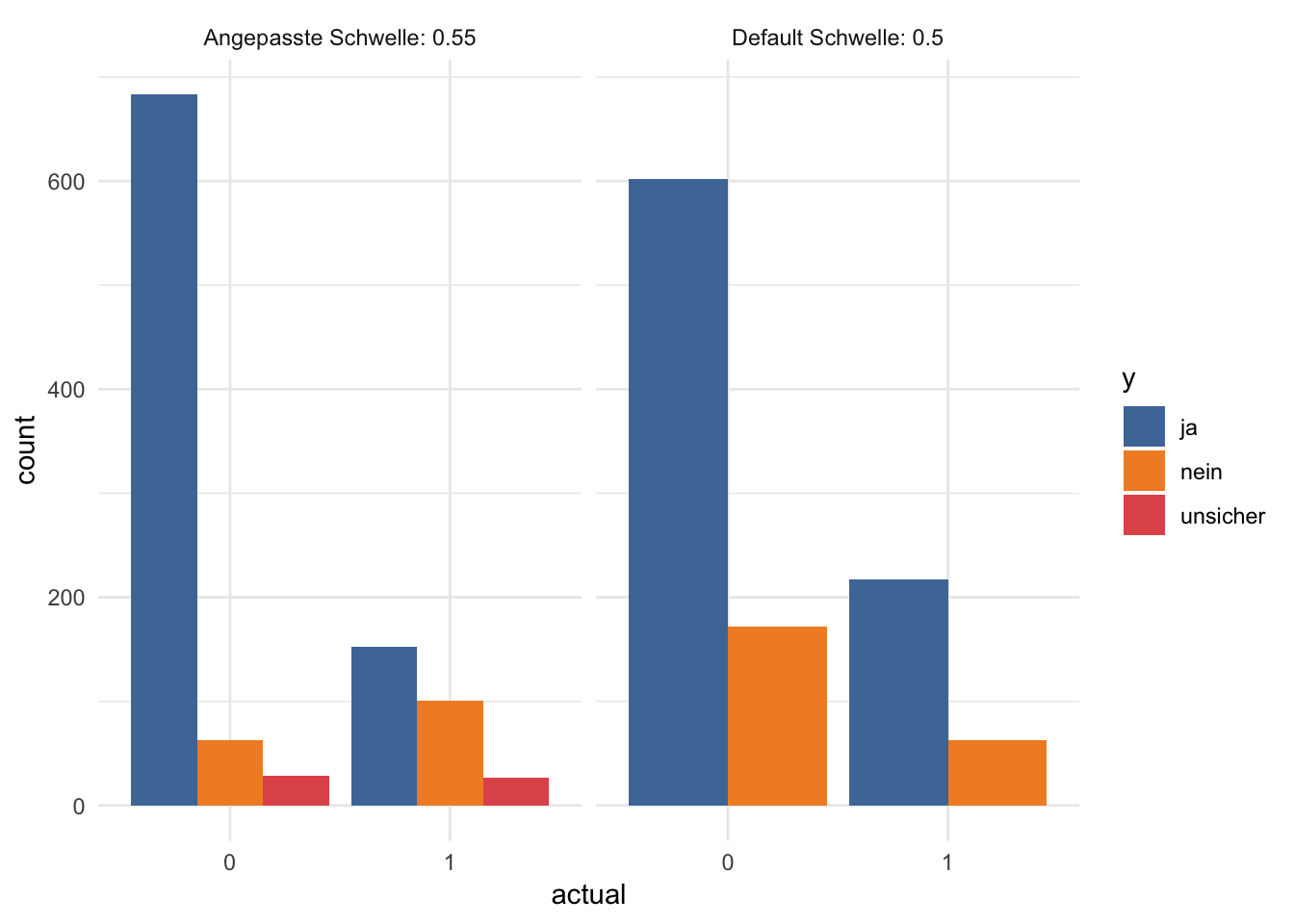``````df <- finalRf_predictions[, c(1, 3, 4)]

thresholds <- seq(from = 0, to = 1, by = 0.1)

prop_table <- data.frame(threshold = thresholds,
prop_p0_true = NA, prop_p0_false = NA,
prop_p1_true = NA, prop_p1_false = NA)

for (threshold in thresholds) {

pred_1 <- ifelse(df\$p1 > threshold, 1, 0)
pred_1_t <- ifelse(pred_1 == df\$actual, TRUE, FALSE)

group <- data.frame(df,
"pred_true" = pred_1_t) %>%
group_by(actual, pred_true) %>%
dplyr::summarise(n = n())

group_p0 <- filter(group, actual == "0")

prop_p0_t <- sum(filter(group_p0, pred_true == TRUE)\$n) / sum(group_p0\$n)
prop_p0_f <- sum(filter(group_p0, pred_true == FALSE)\$n) / sum(group_p0\$n)
prop_table[prop_table\$threshold == threshold, "prop_p0_true"] <- prop_p0_t
prop_table[prop_table\$threshold == threshold, "prop_p0_false"] <- prop_p0_f

group_p1 <- filter(group, actual == "1")

prop_p1_t <- sum(filter(group_p1, pred_true == TRUE)\$n) / sum(group_p1\$n)
prop_p1_f <- sum(filter(group_p1, pred_true == FALSE)\$n) / sum(group_p1\$n)
prop_table[prop_table\$threshold == threshold, "prop_p1_true"] <- prop_p1_t
prop_table[prop_table\$threshold == threshold, "prop_p1_false"] <- prop_p1_f
}``````
``````prop_table %>%
gather(x, y, prop_p0_true, prop_p1_true) %>%
rename(Schwellenwert = threshold) %>%
mutate(x = ifelse(x == "prop_p0_true", "prop true p0",
"prop true p1")) %>%
ggplot(aes(x = Schwellenwert, y = y, color = x)) +
geom_point() +
geom_line() +
scale_color_tableau()``````### Cost/revenue calculation

Let’s assume that

1. a marketing campaign + employee time will cost the company 1000€ per year for every customer that is included in the campaign.
2. the annual average revenue per customer is 2000€ (in more complex scenarios customers could be further divided into revenue groups to calculate how “valuable” they are and how harmful loosing them would be)
3. investing into unnecessary marketing doesn’t cause churn by itself (i.e. a customer who isn’t going to churn isn’t reacting negatively to the add campaign - which could happen in more complex scenarios).
4. without a customer churn model the company would target half of their customer (by chance) for ad-campaigns
5. without a customer churn model the company would lose about 25% of their customers to churn

This would mean that compared to no intervention we would have

• prop_p0_true == customers who were correctly predicted to not churn did not cost anything (no marketing money was spent): +/-0€
• prop_p0_false == customers that did not churn who are predicted to churn will be an empty investment: +/-0€ - 1500€
• prop_p1_false == customer that were predicted to stay but churned: -2000€
• prop_p1_true == customers that were correctly predicted to churn:
• let’s say 100% of those could be kept by investing into marketing: +2000€ -1500€
• let’s say 50% could be kept by investing into marketing: +2000€ * 0.5 -1500€

• Let’s play around with some values:
``````# Baseline
revenue <- 2000
cost <- 1000

customers_churn <- filter(test_data, Churn == 1)
customers_churn_n <- nrow(customers_churn)

customers_no_churn <- filter(filter(test_data, Churn == 0))
customers_no_churn_n <- nrow(customers_no_churn)

customers <- customers_churn_n + customers_no_churn_n

churn_rate_default <- customers_churn_n / customers_no_churn_n
ann_revenue_default <- customers_no_churn_n * revenue

net_win_default``````
``##  1021000``
• How much revenue can we gain from predicting customer churn (assuming conversionr rate of 0.7):
``````conversion <- 0.7

net_win_table <- prop_table %>%
mutate(prop_p0_true_X = prop_p0_true * customers_no_churn_n * revenue,
prop_p0_false_X = prop_p0_false * customers_no_churn_n * (revenue -cost),
prop_p1_false_X = prop_p1_false * customers_churn_n * 0,
prop_p1_true_X = prop_p1_true * customers_churn_n * ((revenue * conversion) - cost)) %>%
group_by(threshold) %>%
summarise(net_win = sum(prop_p0_true_X + prop_p0_false_X + prop_p1_false_X + prop_p1_true_X),
net_win_compared = net_win - net_win_default) %>%
arrange(-net_win_compared)

net_win_table``````
``````## # A tibble: 11 x 3
##    threshold net_win net_win_compared
##        <dbl>   <dbl>            <dbl>
##  1       0.7 1558000           537000
##  2       0.8 1554000           533000
##  3       0.6 1551000           530000
##  4       0.9 1548000           527000
##  5       1   1548000           527000
##  6       0.5 1534000           513000
##  7       0.4 1515600           494600
##  8       0.3 1483400           462400
##  9       0.2 1417200           396200
## 10       0.1 1288200           267200
## 11       0    886000          -135000``````

## LIME

• Explaining predictions
``````Xtrain <- as.data.frame(train_hf)
Xtest <- as.data.frame(test_hf)

# run lime() on training set
explainer <- lime::lime(x = Xtrain,
model = best_model)

# run explain() on the explainer
explanation <- lime::explain(x = Xtest[1:9, ],
explainer = explainer,
n_labels = 1,
n_features = 4,
kernel_width = 0.5)``````
``plot_explanations(explanation)``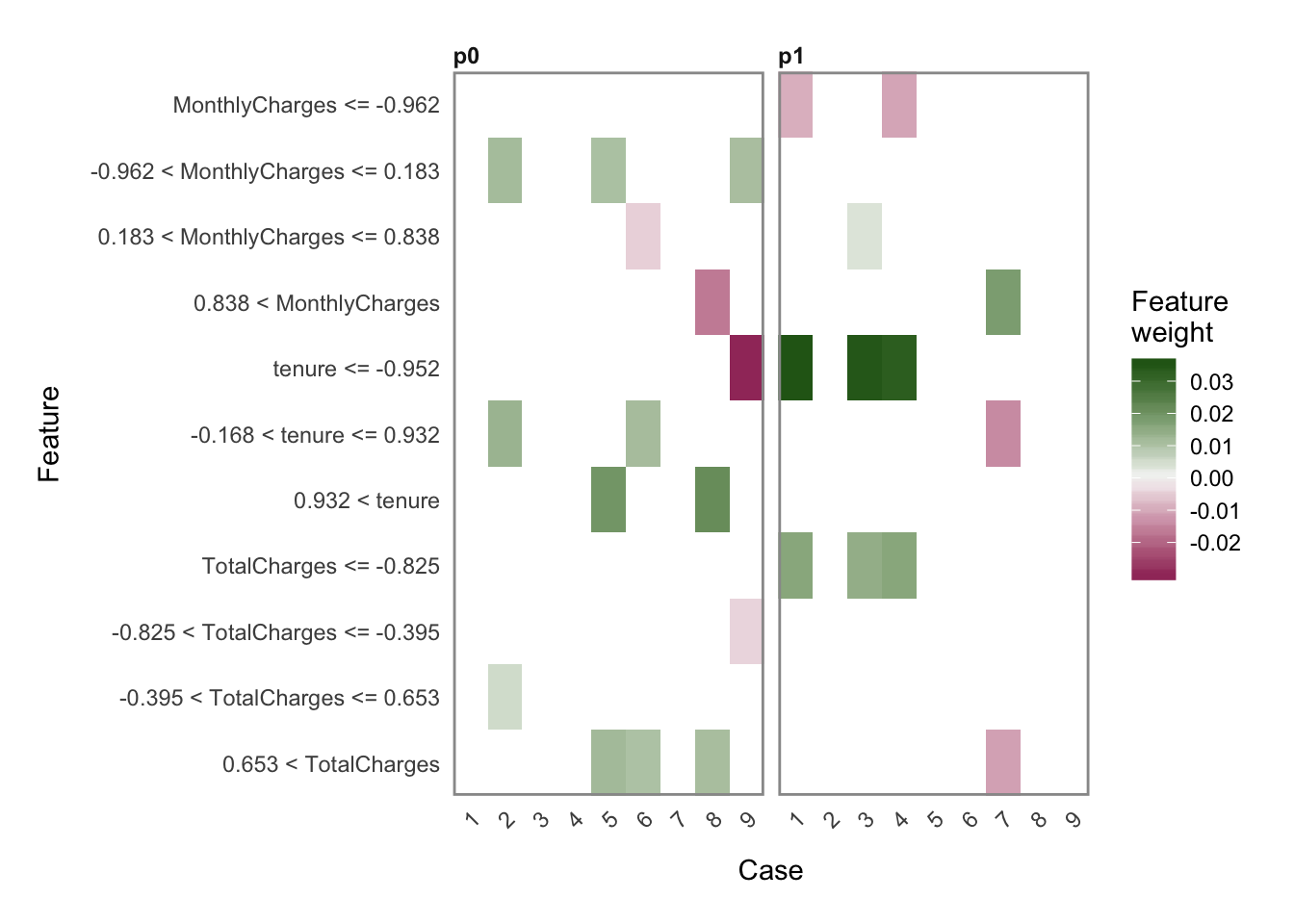``````explanation %>%
plot_features(ncol = 3)``````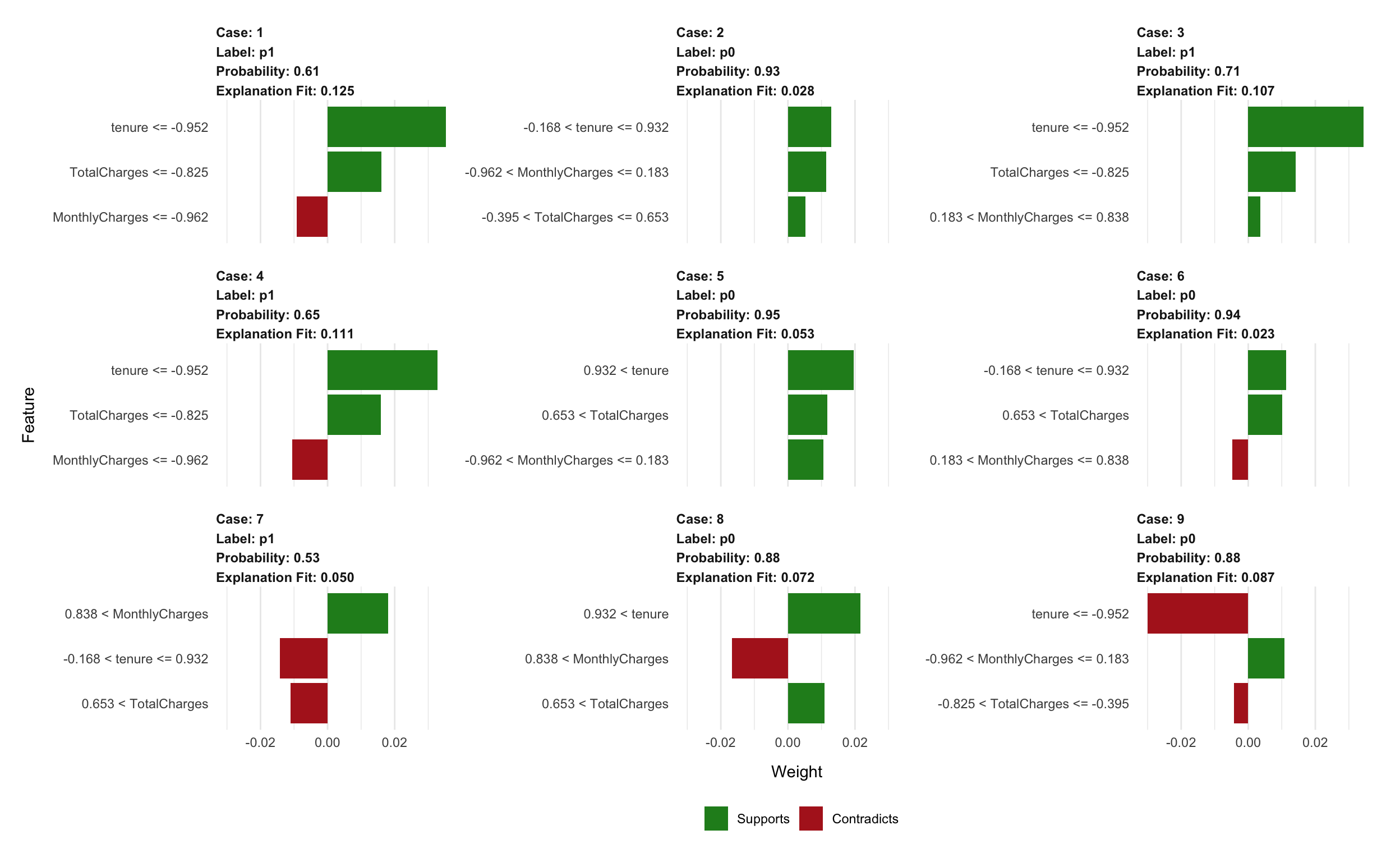``sessionInfo()``
``````## R version 3.5.1 (2018-07-02)
## Platform: x86_64-apple-darwin15.6.0 (64-bit)
## Running under: macOS  10.14.2
##
## Matrix products: default
## BLAS: /Library/Frameworks/R.framework/Versions/3.5/Resources/lib/libRblas.0.dylib
## LAPACK: /Library/Frameworks/R.framework/Versions/3.5/Resources/lib/libRlapack.dylib
##
## locale:
##  en_US.UTF-8/en_US.UTF-8/en_US.UTF-8/C/en_US.UTF-8/en_US.UTF-8
##
## attached base packages:
##  stats     graphics  grDevices utils     datasets  methods   base
##
## other attached packages:
##   h2o_3.20.0.8    bindrcpp_0.2.2  corrplot_0.84   ggthemes_4.0.1
##   yardstick_0.0.2 recipes_0.1.4   rsample_0.0.3   lime_0.4.1
##   keras_2.2.4     mice_3.3.0      caret_6.0-81    lattice_0.20-38
##  forcats_0.3.0   stringr_1.3.1   dplyr_0.7.8     purrr_0.2.5
##  readr_1.3.1     tidyr_0.8.2     tibble_1.4.2    ggplot2_3.1.0
##  tidyverse_1.2.1
##
## loaded via a namespace (and not attached):
##   minqa_1.2.4        colorspace_1.3-2   class_7.3-15
##   base64enc_0.1-3    rstudioapi_0.8     prodlim_2018.04.18
##   fansi_0.4.0        lubridate_1.7.4    xml2_1.2.0
##  codetools_0.2-16   splines_3.5.1      knitr_1.21
##  shinythemes_1.1.2  zeallot_0.1.0      jsonlite_1.6
##  nloptr_1.2.1       pROC_1.13.0        broom_0.5.1
##  tfruns_1.4         shiny_1.2.0        compiler_3.5.1
##  httr_1.4.0         backports_1.1.3    assertthat_0.2.0
##  Matrix_1.2-15      lazyeval_0.2.1     cli_1.0.1
##  later_0.7.5        htmltools_0.3.6    tools_3.5.1
##  gtable_0.2.0       glue_1.3.0         reshape2_1.4.3
##  Rcpp_1.0.0         cellranger_1.1.0   nlme_3.1-137
##  blogdown_0.9       iterators_1.0.10   timeDate_3043.102
##  gower_0.1.2        xfun_0.4           lme4_1.1-19
##  rvest_0.3.2        mime_0.6           pan_1.6
##  MASS_7.3-51.1      scales_1.0.0       ipred_0.9-8
##  hms_0.4.2          promises_1.0.1     parallel_3.5.1
##  yaml_2.2.0         reticulate_1.10    rpart_4.1-13
##  stringi_1.2.4      tensorflow_1.10    foreach_1.4.4
##  lava_1.6.4         bitops_1.0-6       rlang_0.3.0.1
##  pkgconfig_2.0.2    evaluate_0.12      bindr_0.1.1
##  labeling_0.3       htmlwidgets_1.3    tidyselect_0.2.5
##  plyr_1.8.4         magrittr_1.5       bookdown_0.9
##  R6_2.3.0           generics_0.0.2     mitml_0.3-6
##  pillar_1.3.1       haven_2.0.0        whisker_0.3-2
##  withr_2.1.2        RCurl_1.95-4.11    survival_2.43-3
##  nnet_7.3-12        modelr_0.1.2       crayon_1.3.4
##  jomo_2.6-5         utf8_1.1.4         rmarkdown_1.11